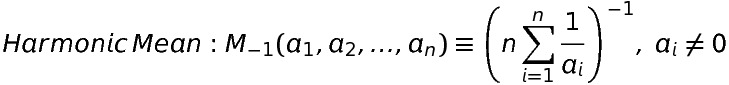iSoul In the beginning is reality.

# Galileo’s method

Extracts about Galileo from Scientific Method: An historical and philosophical introduction by Barry Gower (Routledge, 1997):

Galileo took great pains to ensure that his readers would be persuaded that his conclusions were correct. p. 23

The science of motion was then understood to be a study of the causes of motion, and to be, like any genuine science, a ‘demonstrative’ kind of enquiry. That is to say, experiential knowledge of the facts of motion was superseded by rational knowledge of the causes of those facts, this being accomplished by deductions from fundamental principles, or ‘common notions’, and definitions which were accepted as true. These facts of motion were understood as expressions of common experience rather than as generalisations based upon experiments. This was because the results of the experiments that could be performed were sufficiently uncertain and ambiguous to prevent reliable generalisation; discrepancies between conclusions derived from principles, and experimental results, could be tolerated. The appropriate model of a demonstrative science was Euclidean geometry, where the credibility of a theorem about, say, triangles depends not on how well it fits what we can measure but on its derivability from the basic axioms and definitions of the geometry. p. 23

For Galileo and his contemporaries there was a good reason why demonstration, or proof from first principles, rather than experiment, was required to establish general truths about motion. Any science—scientia—must yield knowledge of what Aristotle had called ‘reasoned facts’, i.e. truths which are both universal and necessary, and such knowledge—philosophical knowledge—can only be arrived at by demonstration. p. 24

there was a long-standing disagreement about the role that mathematics could play in natural philosophy, even though mathematics was able to give certain knowledge. p. 24

In some contexts, notably astronomy and geometry, the more elaborate and intellectually demanding methods of mathematics were often useful and appropriate, but in such contexts it seemed clear that those methods were applicable in so far as what was needed were re-descriptions which could help people formulate accurate predictions. ‘Hypotheses’ which successfully ‘saved the phenomena’, in the sense that they could be used as starting points for derivations of accurate predictions, could meet this need. p. 25

# Mathematics and beauty

Extracts from Scientific Method in Ptolemy’s Harmonics by Andrew Barker (Cambridge University Press 2004):

Mathematics is not the study of all quantities and all quantitative relations indiscriminately. It is the science of beauty. Its task, at the theoretical level, is to interpret, in terms of ‘rationally’ or mathematically intelligible form, the features, movements or states which, when they are present in perceptible phenomena, constitute their aesthetic excellence. p.264

Those of our senses through which we are able to perceive some things as beautiful are therefore involved in an intimate collaboration with mathematical reason. p.264

Since beauty is the manifestation to the senses of that which reason understands as perfect in form, the senses to which beauty is undetectable lack sensitivity, which sight and hearing possess, to those distinctions which, from a rational point of view, are the most significant. p.265

the mathematical sciences have a single objective, the analysis and understanding of the formal basis of beauty p.266

The conception of mathematical science which Ptolemy has presented is that of a capacity that does not merely analyse sets of quantitative relations, but homes in on those that are of special significance, and discovers the principles on which their significance rests. p.268

# Vectors and functions in space and time

A pdf version of this post is here.

The time velocity of an n-dimensional vector variable or vector-valued function Δx per unit of an independent scalar variable t equalsSimilarly, the space lenticity with Δt and Δx, respectively: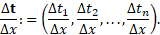The rate of a scalar variable Δx per unit of an independent n-dimensional vector variable or vector-valued function Δt equals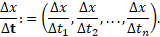The same except with Δt and Δx, respectively: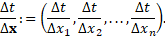# Home is the horizon

As there is an inverse or harmonic algebra, so there is an inverse geometry, an inverse space. Home, the origin, is the horizon, the ends of the earth, and beyond that, the celestial equator, the heavens. We may attempt to journey to the centre of the earth with Jules Verne, but we’ll never make it because it is infinitely far away. We cannot plumb the ultimate depths within, the deep well of the heart. At the centre of it all is a bottomless pit, the hell of eternal darkness.The geometric inverse is with respect to a circle or sphere:

P’ is the inverse of P with respect to the circle. The inverse of the centre is the point at infinity.

The order of events in this geometry is their distance from the horizon, not the centre. The return to home is the end of events, the final event. The later the event, the better, since it is closer to the end, to home.

The destination is where we’ve come from and where we return. It is a round trip, a circuit, a cycle of life and change.

What we call the beginning is often the end
And to make an end is to make a beginning.
The end is where we start from. T. S. Eliot, Little Gidding

# Number and algebra and their dual

For the first post in this series, see here.

(1) Set theory and logic, (2) number and algebra, and (3) space and time are three foundational topics that have dual approaches. Let us begin with the standard approaches to these three topics, and then define duals to each of them. In some ways, the original and the dual may be used together.

(2) Number and algebra

The concept of counting and number is as universal as language, though the full definition of number did not occur until the 19th century. Algebra came to the West from India and Arabia in the Middle Ages but its formal definition did not occur until the 19th century. Abstract algebra also began in the 19th century.

The basic rules of algebra are as follows: addition and multiplication are commutative and associative; multiplication distributes over addition; addition and multiplication have identities and inverses with one exception: there is no multiplicative inverse for zero.

An idea of infinity comes from taking the limit of a number as its value approaches zero: ∞ ∼ 1/x as x → 0. Infinity can be partially incorporated via limits.

Dual: harmonic numbers

An additive dual can be defined by negating every number. A more interesting dual comes from taking the multiplicative dual of every number. This latter case can be called harmonic numbers and harmonic algebra because of its relation to the harmonic mean.

The harmonic isomorphism relates every number x to its harmonic dual by H(x) := 1/y. The dual of zero is ∞.

For harmonic algebra: see here.

Harmonic algebra is the multiplicative inverse of ordinary algebra. There is a sense in which harmonic algebra counts down rather than up. Zero in harmonic numbers is like infinity in ordinary numbers. Larger harmonic numbers correspond to smaller ordinary numbers. Smaller harmonic numbers correspond to larger ordinary numbers.

# Set theory and logic and their dual

(1) Set theory and logic, (2) number and algebra, and (3) space and time are three foundational topics that each have duals. Let us begin with the standard approaches to these three topics, and then define duals to each of them. To some extent, the original and the dual may be used together.

(1) Set theory and logic

A set is defined by its elements or members. Its properties may also be known or specified, but what is essential to a set is its members, not its properties. The notation for “x is an element of set S” is “x ∈ S”. A subset is a set whose members are all within another set: “s is a subset of S” is “s ⊆ S”. If subset s does not (or cannot) equal S, then it is a proper subset: “s ⊂ S”.

The null set (∅) is a unique set defined as having no members. That is paradoxical but not contradictory. A universal set (Ω) is defined as having all members within a particular universe. An unrestricted universal set is not defined because it would lead to contradictions.

The complement of a set (c) is the set of all elements within a particular universe that are not in the set. A union (∪) of sets is the set containing all members of the referenced sets. An intersection (∩) of sets is defined as the set whose members are contained in every referenced set.

Set theory has a well-known correspondence with logic: negation (¬) corresponds to complement, disjunction (OR, ∨) corresponds to union, and conjunction (AND, ∧) corresponds to intersection. Material implication (→) corresponds to “is a subset of”. Contradiction corresponds to the null set, and tautology corresponds to the universal set.

# Harmonic algebra

This revised post follows up on harmonic addition mentioned in a previous post here.

Harmonic algebra is an inverse algebra. It is based on an automorphism that interchanges the zero with the infinite and the greater-than-one with the less-than-one: 0 ↔ ∞ and x ↔ 1/x. So zero becomes the new inaccessible number and infinity becomes the new additive unit. That is,

xy := g−1(g(x) + g(y))

xy := g−1(g(x) ∙ g(y))

etc., where g(x) = 1/x with x ≠ 0.

Regular and harmonic algebra are isomorphic with ordinary addition exchanged with harmonic multiplication and ordinary multiplication exchanged with harmonic division. Harmonic algebra is like counting down from infinity, in which an increment of one reduces the amount slightly.

Harmonic addition is defined as a power operation:

with the understanding that (1/0) → ∞ and (1/∞) → 0. An intermediate value may be zero but not a final value.

Simple harmonic addition is thus defined as:

# Combining equations

Given two equations with the same variable, how can they be combined? If the equations are consistent, they may be solved as simultaneous equations. But what if the equations are inconsistent? There are two ways to combine them in that case, one is OR, the other is AND.

Consider the equations x = a and x = b, where a ≠ b. If we multiply these equations together, we get

x² = ab,

in which the solution is x = √ab, so that x is the geometric mean of a and b.

If we make the equations homogeneous first, then multiply them together, we get: 0 = x − a and 0 = x − b, so that

0 = (x − a) (x − b) = x² − (a + b) x + ab.

The solution of the combined equation is either x = a or x = b. To combine equations with AND, multiply homogeneous equations together.

Another way to combine equations is to add them together. In this case, we get

x + x = 2x = a + b, or x = (a + b)/2,

so that x is the arithmetic mean of a and b. Homogeneous equations added produce the same result: 0 = x − a + x − b = 2x − (a + b), so that x = (a + b)/2.

# Elemental inverse

Begin with elements. Elements are a very general concept: they may be either members of sets or distinctions of classes. As a set is defined by its members, so a class is defined by its distinctions. So, the elements of sets are members and the elements of classes are distinctions.

Sets may be divided into subsets or combined into supersets. Classes may be divided into subclasses or combined into superclasses. Distinctions may be between classes or within classes. Members may be within sets or without sets.

One might say that a class is just a set of distinctions, or one might also say that a set is just a class of members. But that would blur their differences.

Sets assume one knows members and is trying to combine them into the right sets. Classes assume one knows distinctions and is trying to divide them into the right classes. Aristotle assumed that classes could be known by defining them with the right distinctions. Empiricists assume that sets can be known by defining them with the right members.

Realists begin with classes. A tree is defined by its distinctions. Upon inductive investigation, trees may be grouped into types of tree. Upon deductive investigation, types of trees have certain properties.

Induction proceeds from classes to sets. Deduction proceeds from sets to classes. Sets and classes are like inverses of one another.

Both sets and classes are axiomatized by Boolean algebra with the axioms of identity, complementation, associativity, commutativity, and distributivity.

# Means and operations

The power means are defined for a set of real numbers, a1, a2, …, an: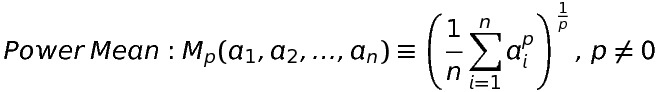The best-known of these are the arithmetic, geometric, and harmonic means, with p = 1, p = –1, and  p → 0: